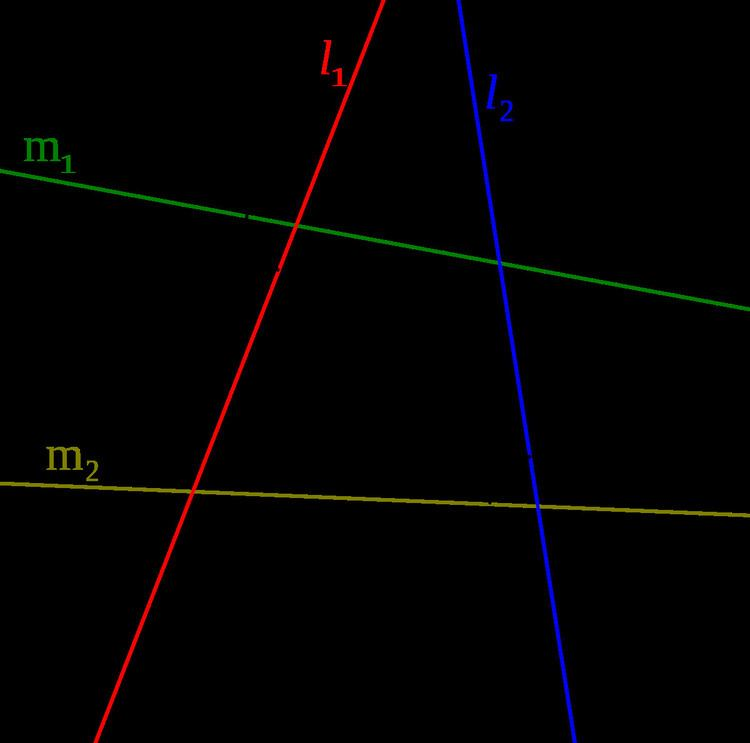# Antiparallel (mathematics)

Updated on
Covid-19In geometry, anti-parallel lines can be defined with respect to either lines or angles.

## Definitions

Given two lines m 1 and m 2 , lines l 1 and l 2 are anti-parallel with respect to m 1 and m 2 if 1 = 2 in Fig.1. If l 1 and l 2 are anti-parallel with respect to m 1 and m 2 , then m 1 and m 2 are also anti-parallel with respect to l 1 and l 2 .

In any quadrilateral inscribed in a circle, any two opposite sides are anti-parallel with respect to the other two sides (Fig.2).

Two lines l 1 and l 2 are antiparallel with respect to the sides of an angle if and only if they make the same angle A P C in the opposite senses with the bisector of that angle (Fig.3).

## Antiparallel vectors

In a Euclidean space, two directed line segments, often called vectors in applied mathematics, are antiparallel, if they are supported by parallel lines and have opposite directions. In that case, one of the associated Euclidean vectors is the product of the other by a negative number.

## Relations

1. The line joining the feet to two altitudes of a triangle is antiparallel to the third side.(any cevians which 'see' the third side with the same angle create antiparallel lines)
2. The tangent to a triangle's circumcircle at a vertex is antiparallel to the opposite side.
3. The radius of the circumcircle at a vertex is perpendicular to all lines antiparallel to the opposite sides.

Similar Topics